# S Block - Group 2 Questions and Answers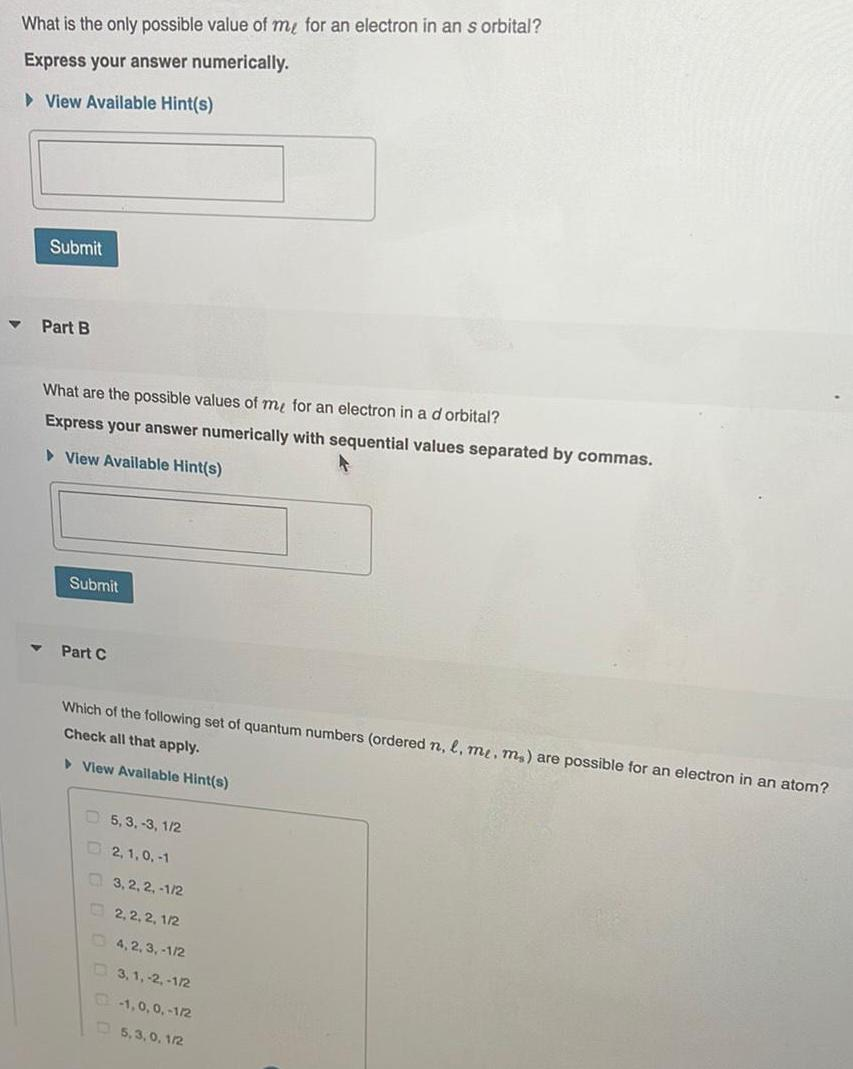Inorganic Chemistry
S Block - Group 2
What is the only possible value of me for an electron in an s orbital? Express your answer numerically. Part B What are the possible values of me for an electron in a d orbital? Express your answer numerically with sequential values separated by commas. Which of the following set of quantum numbers (ordered n, l, me, m.) are possible for an electron in an atom? Check all that apply. 5, 3, -3, 1/2 2,1,0,-1 3, 2, 2, -1/2 2, 2, 2, 1/2 4,2,3, -1/2 3,1, -2, -1/2 -1,0,0,-1/2 5,3,0, 1/2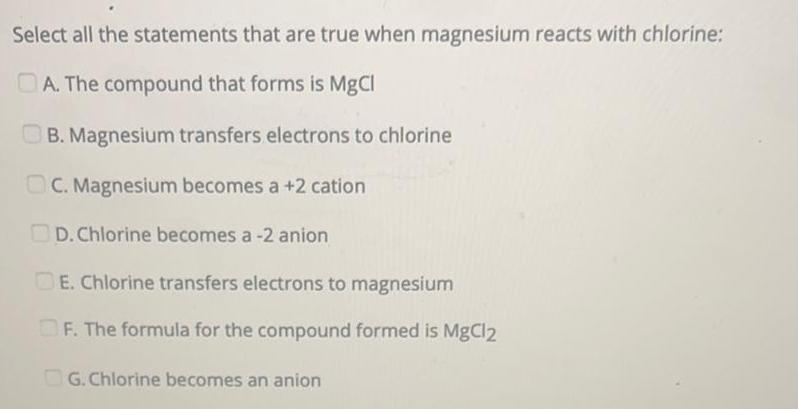Inorganic Chemistry
S Block - Group 2
Select all the statements that are true when magnesium reacts with chlorine: A. The compound that forms is MgCl B. Magnesium transfers electrons to chlorine C. Magnesium becomes a +2 cation D. Chlorine becomes a -2 anion DE. Chlorine transfers electrons to magnesium F. The formula for the compound formed is MgCl2 G. Chlorine becomes an anion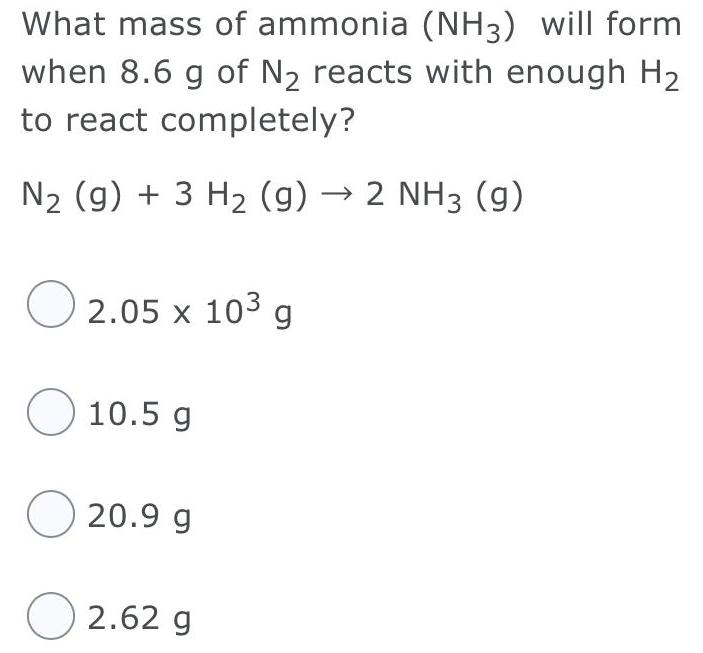Inorganic Chemistry
S Block - Group 2
What mass of ammonia (NH3) will form when 8.6 g of N₂ reacts with enough H₂ to react completely? N₂ (g) + 3 H₂ (g) → 2 NH3 (9) 2.05 x 10³ g 10.5 g 20.9 g 2.62 g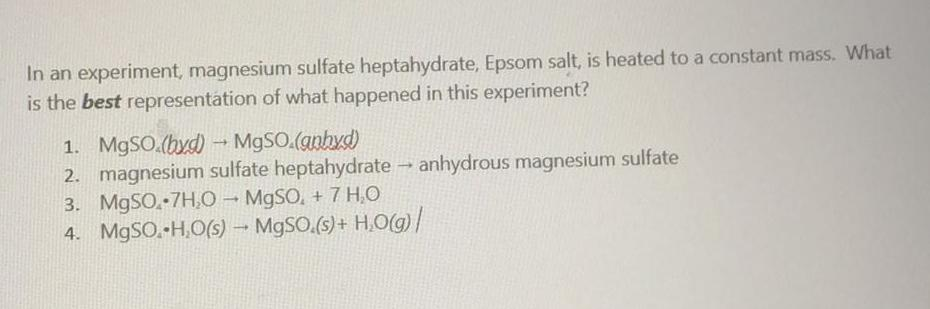Inorganic Chemistry
S Block - Group 2
In an experiment, magnesium sulfate heptahydrate, Epsom salt, is heated to a constant mass. What is the best representation of what happened in this experiment? 1. MgSO4 (byd) → MgSO4(gnhyd) 2. magnesium sulfate heptahydrate → anhydrous magnesium sulfate 3. MgSO.7H₂O → MgSO, + 7 H₂O 4. MgSO.H₂O(s) → MgSO.(s) + H₂O(g)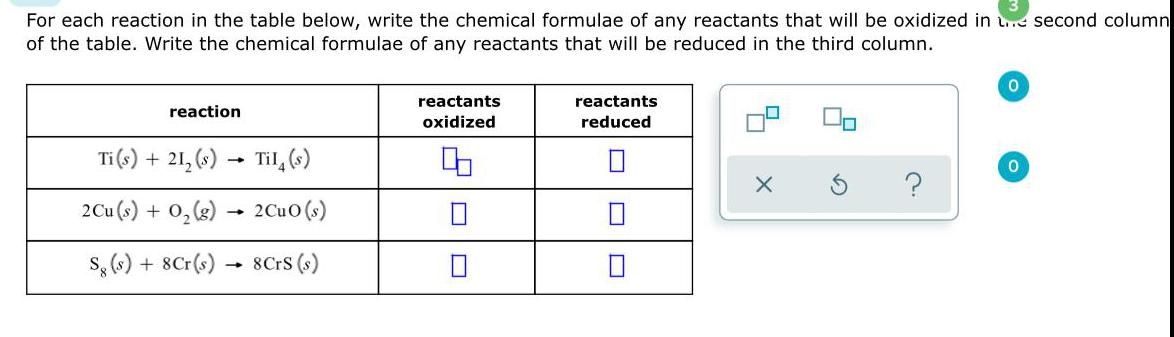Inorganic Chemistry
S Block - Group 2
For each reaction in the table below, write the chemical formulae of any reactants that will be oxidized in second column of the table. Write the chemical formulae of any reactants that will be reduced in the third column.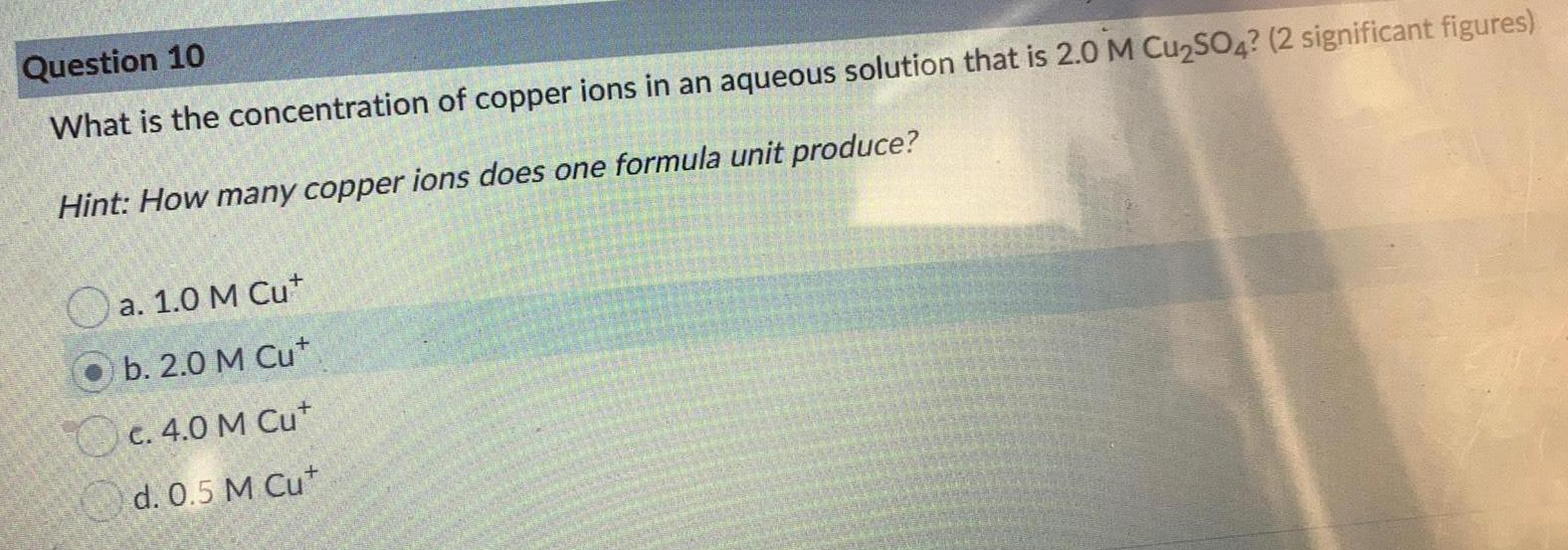Inorganic Chemistry
S Block - Group 2
What is the concentration of copper ions in an aqueous solution that is 2.0 M Cu₂SO4? (2 significant figures) Hint: How many copper ions does one formula unit produce? a. 1.0 M Cu b. 2.0 M Cut c. 4.0 M Cu+ d. 0.5 M Cu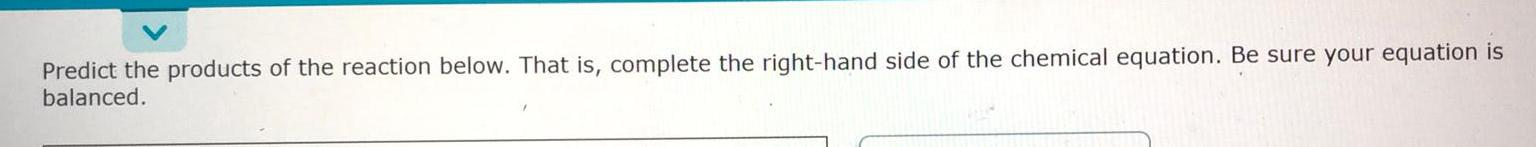Inorganic Chemistry
S Block - Group 2
Predict the products of the reaction below. That is, complete the right-hand side of the chemical equation. Be sure your equation is balanced.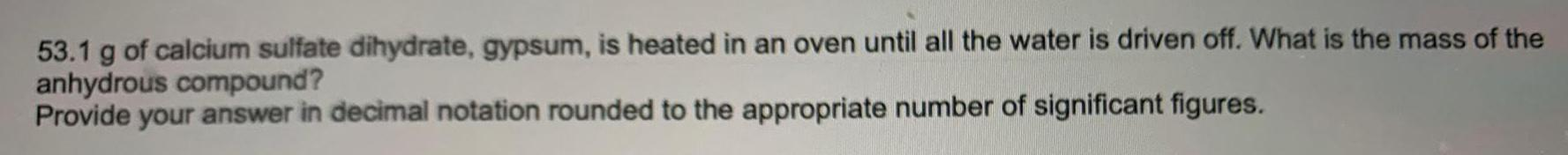Inorganic Chemistry
S Block - Group 2
53.1 g of calcium sulfate dihydrate, gypsum, is heated in an oven until all the water is driven off. What is the mass of the anhydrous compound? Provide your answer in decimal notation rounded to the appropriate number of significant figures.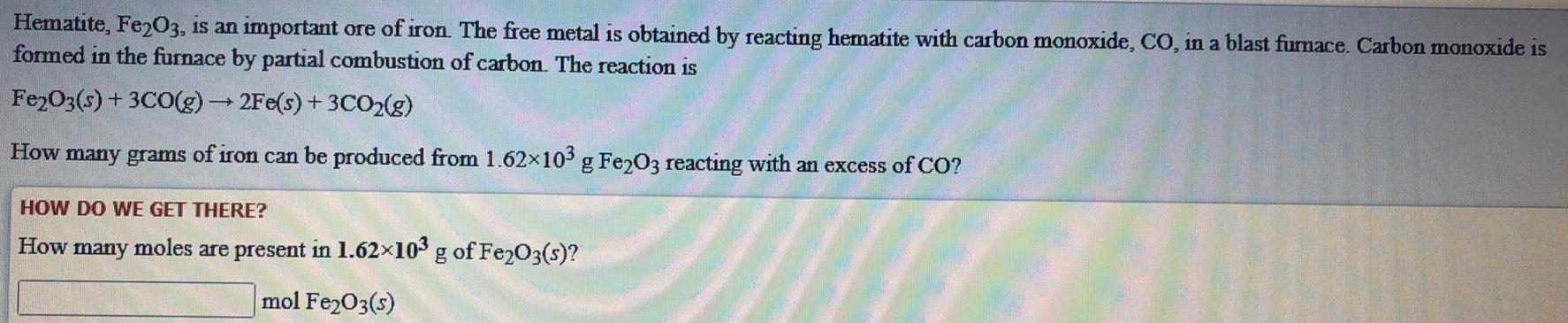Inorganic Chemistry
S Block - Group 2
Hematite, Fe2O3, is an important ore of iron. The free metal is obtained by reacting hematite with carbon monoxide, CO, in a blast furnace. Carbon monoxide is formed in the furnace by partial combustion of carbon. The reaction is Fe2O3(s) + 3CO(g) → 2Fe(s) + 3CO2(g) How many grams of iron can be produced from 1.62×103 g Fe2O3 reacting with an excess of CO? HOW DO WE GET THERE? How many moles are present in 1.62×10³ g of Fe₂O3(s)? mol Fe2O3(s)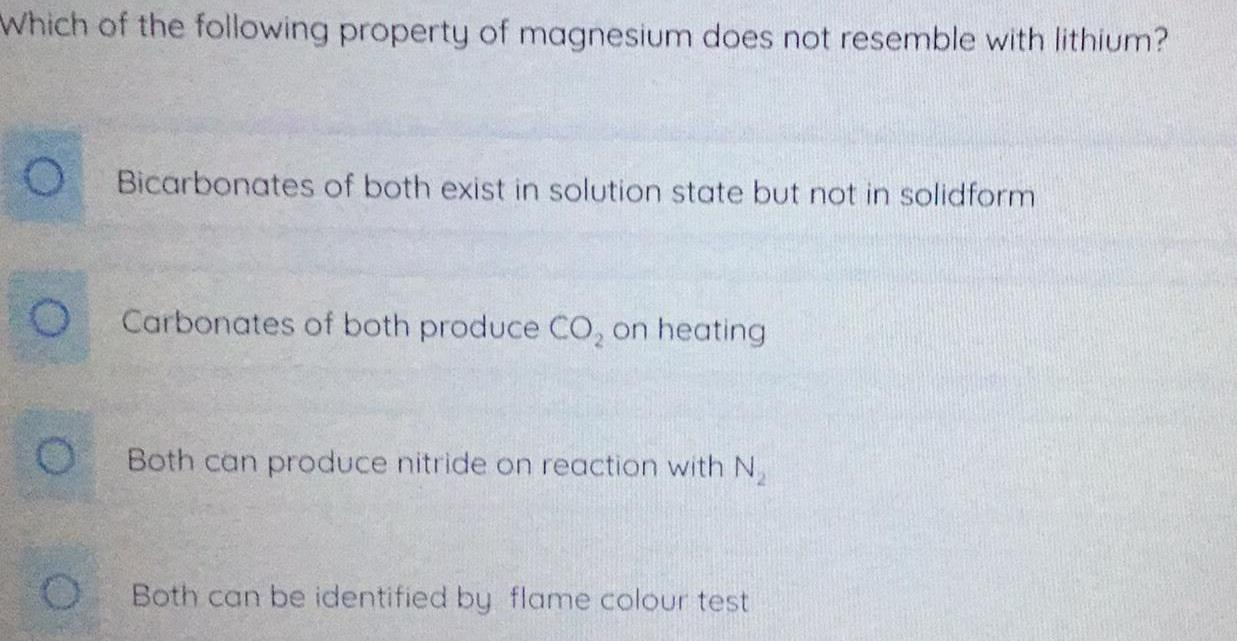Inorganic Chemistry
S Block - Group 2
Which of the following property of magnesium does not resemble with lithium? Bicarbonates of both exist in solution state but not in solidform Carbonates of both produce CO₂ on heating Both can produce nitride on reaction with N₂ Both can be identified by flame colour testInorganic Chemistry
S Block - Group 2
The first ionization energy of Mg is 735 kJ/mol. The second ionization energy is: less than 735 kJ/mol 735 kJ/mol More information is needed to answer this question. greater than 735 kJ/mol None of these choices are correct.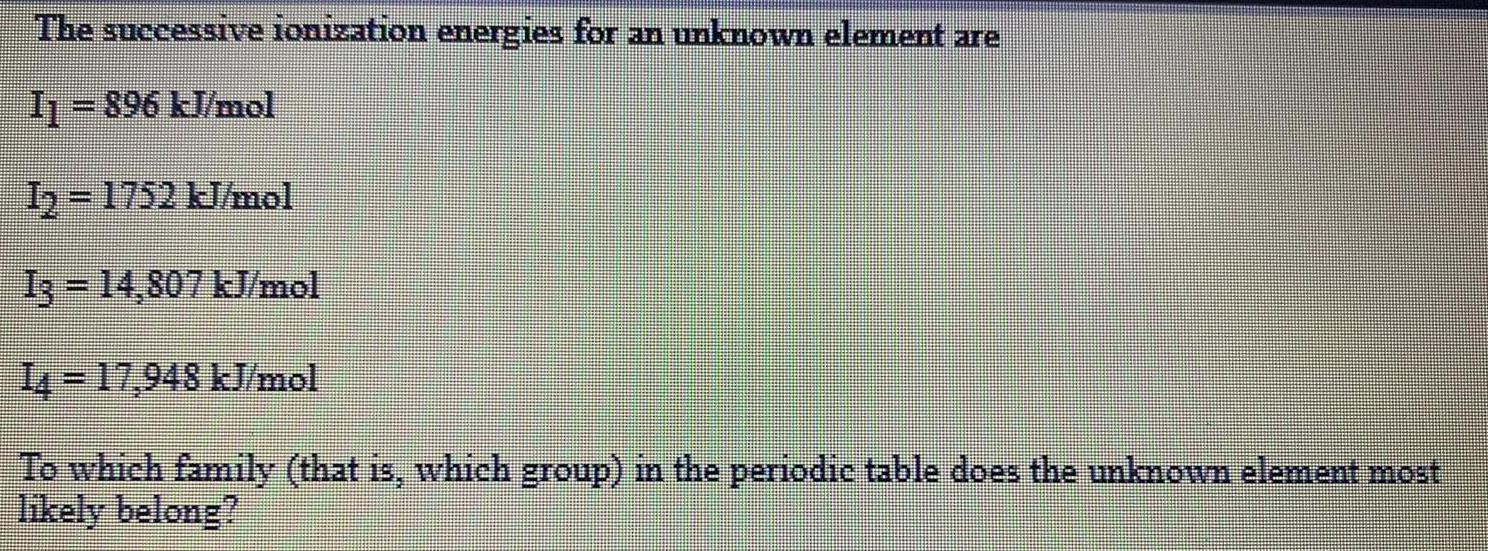Inorganic Chemistry
S Block - Group 2
The successive ionization energies for an unknown element are I₁ = 896 kJ/mol 1₂-1752 kJ/mol 13-14,807 kJ/mol 14-17,948 kJ/mol To which family (that is, which group) in the periodic table does the unknown element most likely belong?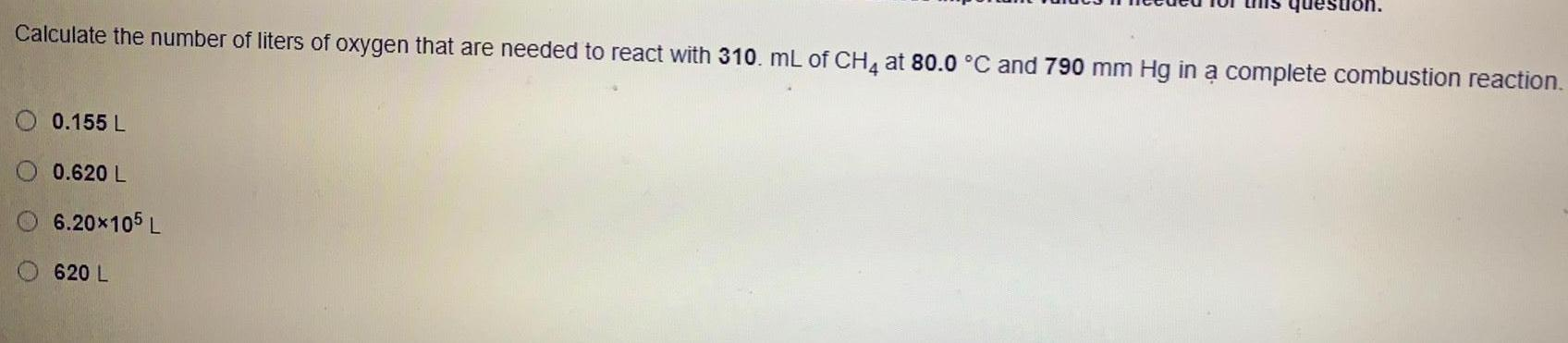Inorganic Chemistry
S Block - Group 2
Calculate the number of liters of oxygen that are needed to react with 310. mL of CH4 at 80.0 °C and 790 mm Hg in a complete combustion reaction. 0.155 L 0.620 L 6.20×10^5 L 620 L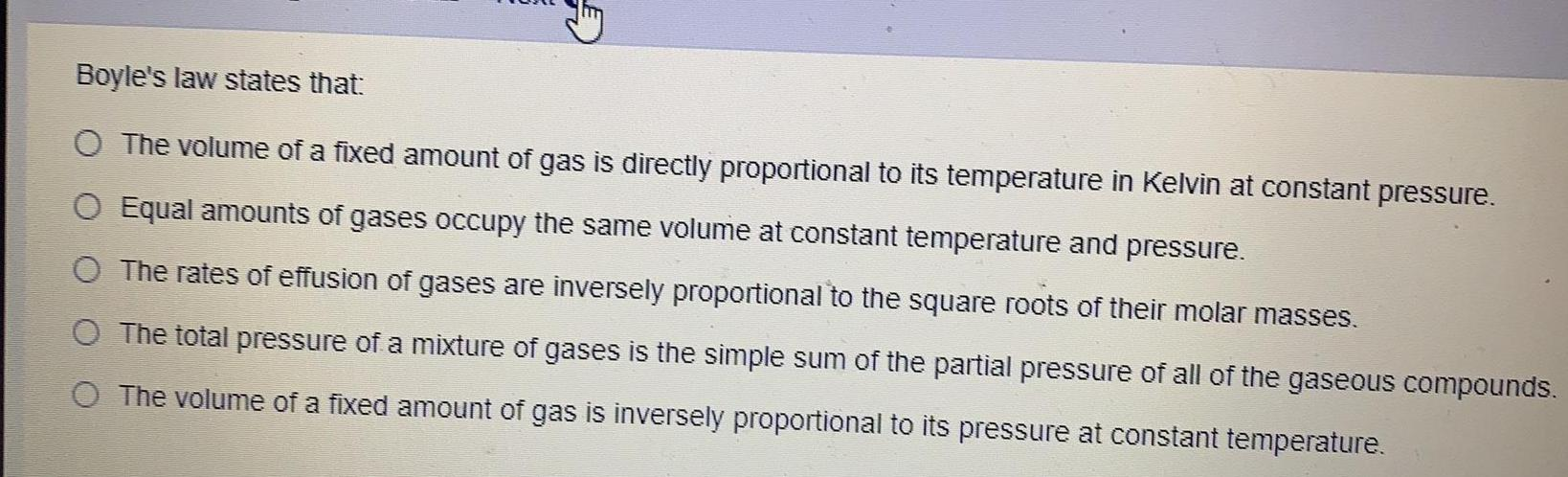Inorganic Chemistry
S Block - Group 2
Boyle's law states that: The volume of a fixed amount of gas is directly proportional to its temperature in Kelvin at constant pressure. Equal amounts of gases occupy the same volume at constant temperature and pressure. The rates of effusion of gases are inversely proportional to the square roots of their molar masses. The total pressure of a mixture of gases is the simple sum of the partial pressure of all of the gaseous compounds. The volume of a fixed amount of gas is inversely proportional to its pressure at constant temperature.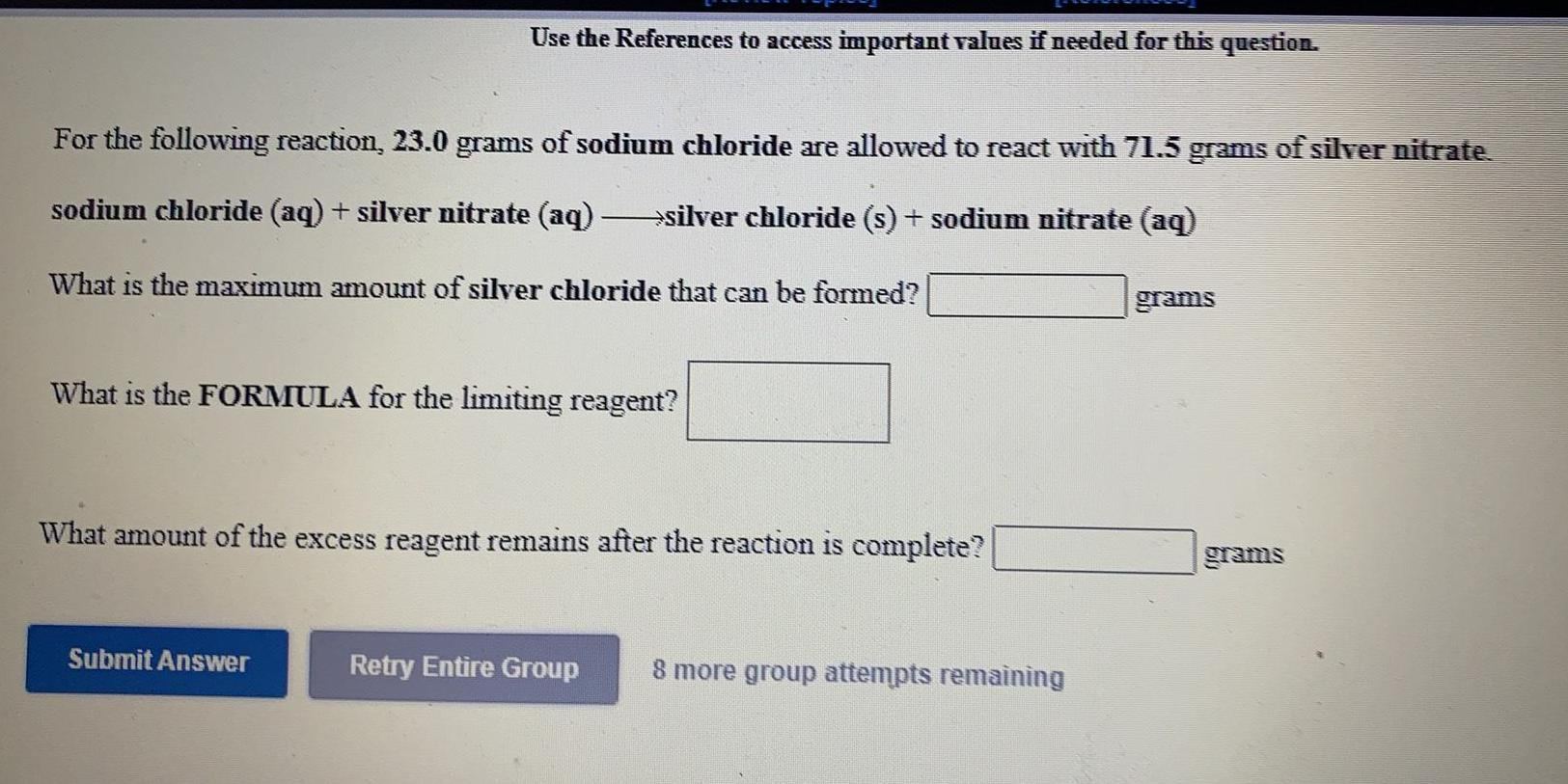Inorganic Chemistry
S Block - Group 2
For the following reaction, 23.0 grams of sodium chloride are allowed to react with 71.5 grams of silver nitrate. sodium chloride (aq) + silver nitrate (aq) →silver chloride (s) + sodium nitrate (aq) What is the maximum amount of silver chloride that can be formed? What is the FORMULA for the limiting reagent? What amount of the excess reagent remains after the reaction is complete?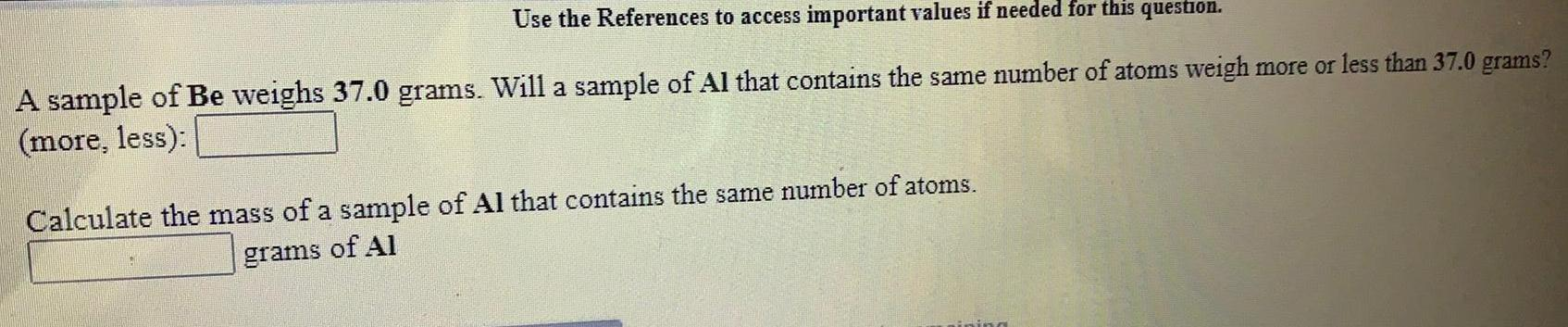Inorganic Chemistry
S Block - Group 2
.A sample of Be weighs 37.0 grams. Will a sample of Al that contains the same number of atoms weigh more or less than 37.0 grams? (more, less):_________ Calculate the mass of a sample of Al that contains the same number of atoms _______grams of Al